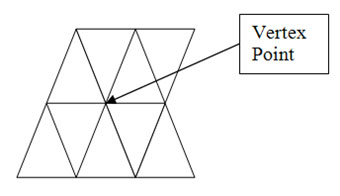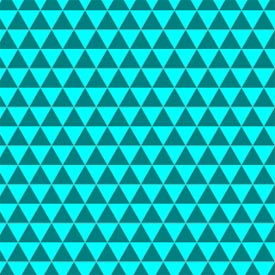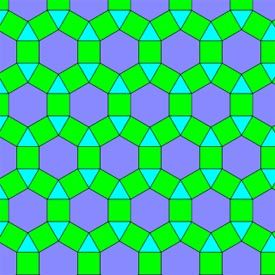What are Tessellations?

# What are Tessellations?

Patterns are one aspect of geometry which are frequently found and utilized. From kindergarten throughout one's schooling the use of patterns and their importance are reviewed. Being able to recognize patterns is an important concept. Often patterns are represented using capital letters. For example, there is the simple AB pattern. In the AB pattern, two different colors or objects are used to create the pattern. These two different items are repeated in an every other one format. An example of an AB pattern using colors would be the following: purple, blue, purple, blue, purple, blue, etc... An example of an AB pattern using objects might be the following: square, circle, square, circle, square, circle, etc... Patterns of this type continue on indefinitely as long as the pattern continues to repeat.

Patterns can be simple as in the above example, or more complex. An ABC pattern involves three repetitions such as red, blue, yellow, red, blue, yellow. Sometimes the same object or color is used more than once in a pattern. In this case, the pattern uses the same letter to represent both uses. An example of this would be an ABB pattern. In this case it might look like: square, circle, circle, square, circle, circle, etc... Notice that the two circles are both represented by the label of B. The letters used to describe the pattern allow for a common understanding of the information provided. Describing a variety of patterns using this format provides a better understanding to all involved.

A tessellation is defined as a pattern of shapes which are all closed and totally cover a surface. Tessellations do not need to be patterns as those described in the above paragraphs. Tessellations do not need to repeat in any of the ways previously described. The most common places where you will see tessellations are on floors and walls. When you look at a tiled surface, you are looking at an example of a tessellation. There are no shapes which overlap and there are no gaps between the shapes. In some cases, you will find tessellations which are created by repeated patterns. However, in other cases you will find pictures or designs created within a tessellation, which in no way repeat. An example of a tessellation which could be created by shows no repetition would include a mosaic picture. This picture, created by tiles, would not necessarily repeat. Instead, the tiles would combine to form one larger picture. Both, with repetitions or without repetitions, of the tiled surfaces would be considered tessellations. Keeping in mind that they are created with shapes which are closed and there are no gaps and the fact that the entire surface is covered, it will be considered a tessellation.

The point or corner of a shape is referred to as the vertex (vertices is the plural). It is important to understand the vertex in relation to a tessellation. As the points must not overlap or leave gaps, the points will touch in some manner. The touching of points is critical to the completion of a tessellation. As the shapes must touch leaving no gaps or overlaps in order to create a tessellation, often they will meet at the vertex. Consider even two squares that are side by side with both sides fully touching. Even in such a situation, the corners or vertices will also touch.

Since there will be no gaps or overlaps, there are some shapes which are more difficult to use in a tessellation than others. The regular shapes, such as a square, triangle or rectangle, can easily be incorporated into a tessellation. This would explain why many floor tiles or ceramic tile patterns involve some of these more basic shapes. Circles would be quite difficult to incorporate into a tessellation, thus the rarity of circles in ceramic tiles or in flooring patterns.

In a tessellation, the point where the shapes come together is known as the vertex point. The vertex point is the sum of all of the angles where the points of each shape come together and will equal exactly three hundred sixty degrees. If the sum is more than three hundred sixty degrees, the shapes will overlap and if it is less than three hundred sixty degrees, there will be gaps. At this point, measuring the angles is critical, simply having an understanding that there can be no gaps or overlaps. See the example below:

Want to learn more? Take an online course in Geometry.There are two types of tessellations: regular and semiregular.A regular tessellation is created when congruent copies of only one regular polygon are used to form the pattern. In other words, the same sized shape is used over and over to create the pattern which covers the surface. Additionally, three of the vertices and three angles of the regular polygon will meet at each vertex point. In a regular tessellation, all of the vertex points will look exactly the same. This is due to the fact that only congruent copies of a regular polygon are used to create the tiled pattern. Regular tessellations could be continued on forever with the tiling pattern continually being repeated.

Some examples of shapes which can be used to create a regular tessellation follow:

Equilateral triangles can be used to create a regular tessellation because the interior angles of each triangle are sixty degrees. Six of these sixty degree angles come together at each vertex point creating the necessary three hundred and sixty degree angles. As there are no gaps or overlaps, a true regular tessellation is created.

Squares can also be used to create a regular tessellation as the interior angle is measured at ninety degrees. Four of these ninety degree angles touch at each vertex point which then creates the necessary three hundred sixty degree angles.

Hexagons can also be used to create a regular tessellation. The angle measurement for a regular hexagon is one hundred twenty degrees. As three come together at each vertex point, the necessary three hundred sixty degrees is created.

Notice that a shape such as a pentagon would not be able to be used to create a regular tessellation. In the case of the pentagon, the angles measure at one hundred eight degrees. Three come together creating only three hundred twenty four degrees at the vertex point. This does not meet the criteria of three hundred sixty degrees and would in-fact leave significant gaps throughout the pattern.A semiregular tessellation utilizes more than one shape. The rules involved in semiregular tessellation are:
• Must use two or more different shapes
• All of the shapes used must be regular polygons
• The pattern must continue in the same order as they meet at the vertex point

Using examples from the above described regular tessellations, one can predict which shapes can be successfully combined to create a semiregular tessellation. Triangles and squares or hexagons and triangles are two examples of regular polygons which can be combined to form a semiregular tessellation. Keep in mind that in order for a semiregular tessellation to be a true tessellation, the vertex points must remain the same throughout the design. Therefore, not all created patterns using hexagons and triangles will in fact represent a true semiregular tessellation.

Naming a semiregular tessellation can be done by looking closely at one vertex point. Working your way around the vertex point, beginning with the polygon with the smallest number of sides, count the number of sides of each polygon at each vertex point. The label or name of the semiregular tessellation then becomes these numbers. An example might be something such as: 3,3,3,3,6 for a tessellation created using triangles and hexagons. Notice that in this example, four triangles and one hexagon would all meet at each vertex point.

Tessellations can be utilized to create puzzles, art, patterns and numerous other things. There are some very famous artists and mathematicians whose work is based on the concept of tessellations. In this way, artists use pictures of animals or other shapes to fill their canvas, while still meeting the requirement that the pictures do not overlap or leave any gaps. M.C. Esher is quite famous for his work involving tessellations. Esher uses a variety of non-traditional shapes and lines to create his tessellations. This makes them quite unique as well as great works of art.

Exercises:

1. Create a tessellation of your choice using your word processing program. Label the tessellation as regular or semiregular.

2. Describe why a certain shape would be difficult to use to
create a tessellation.

Explore